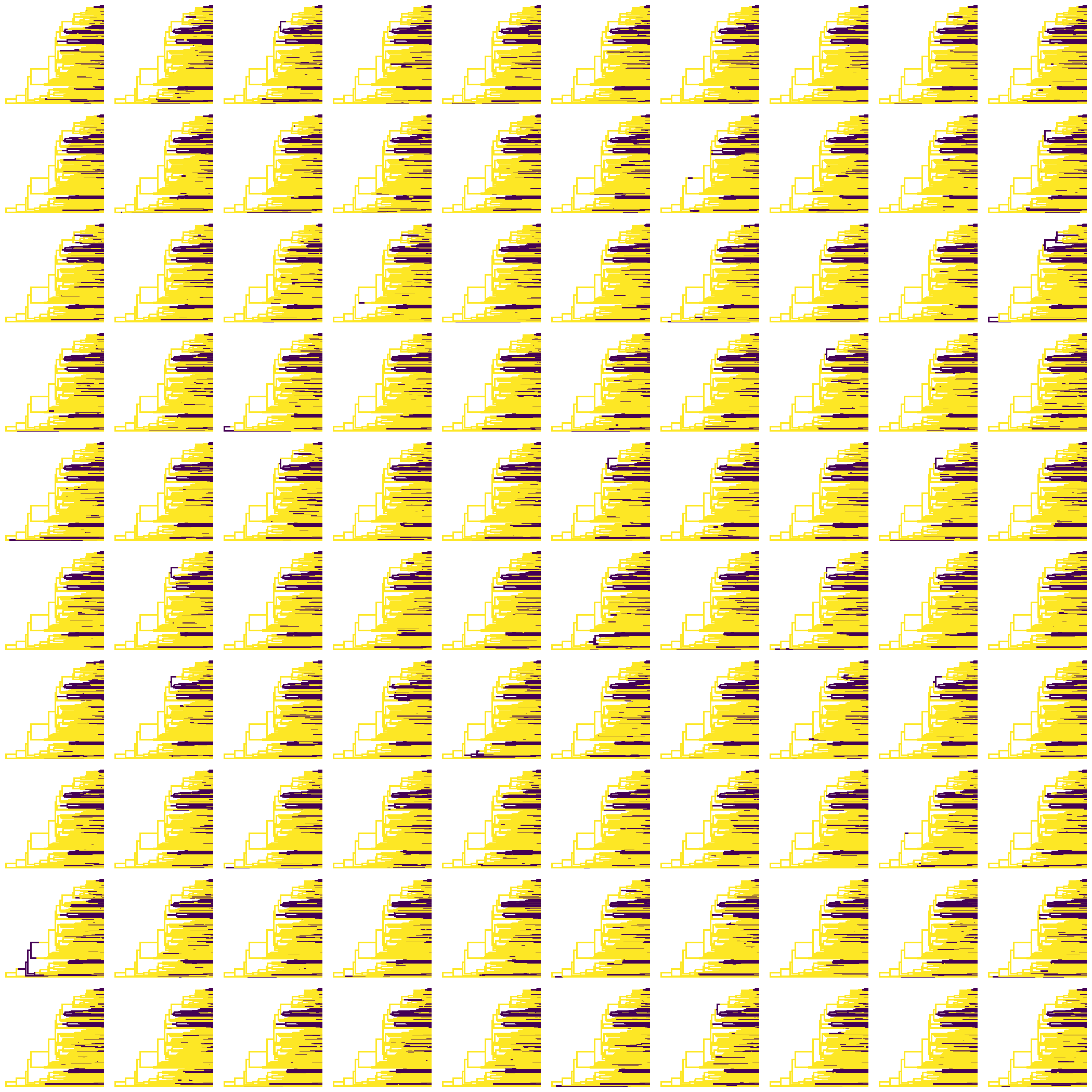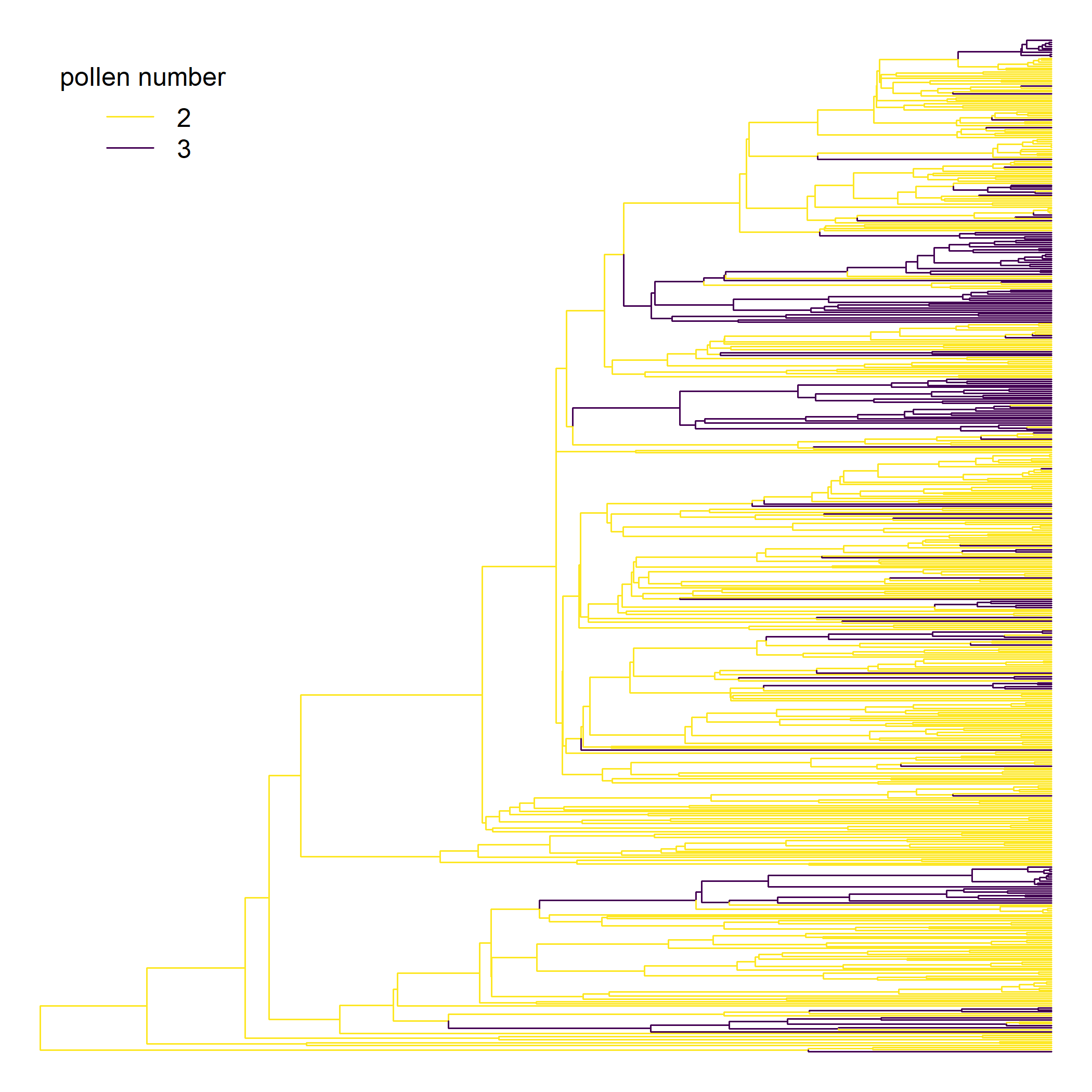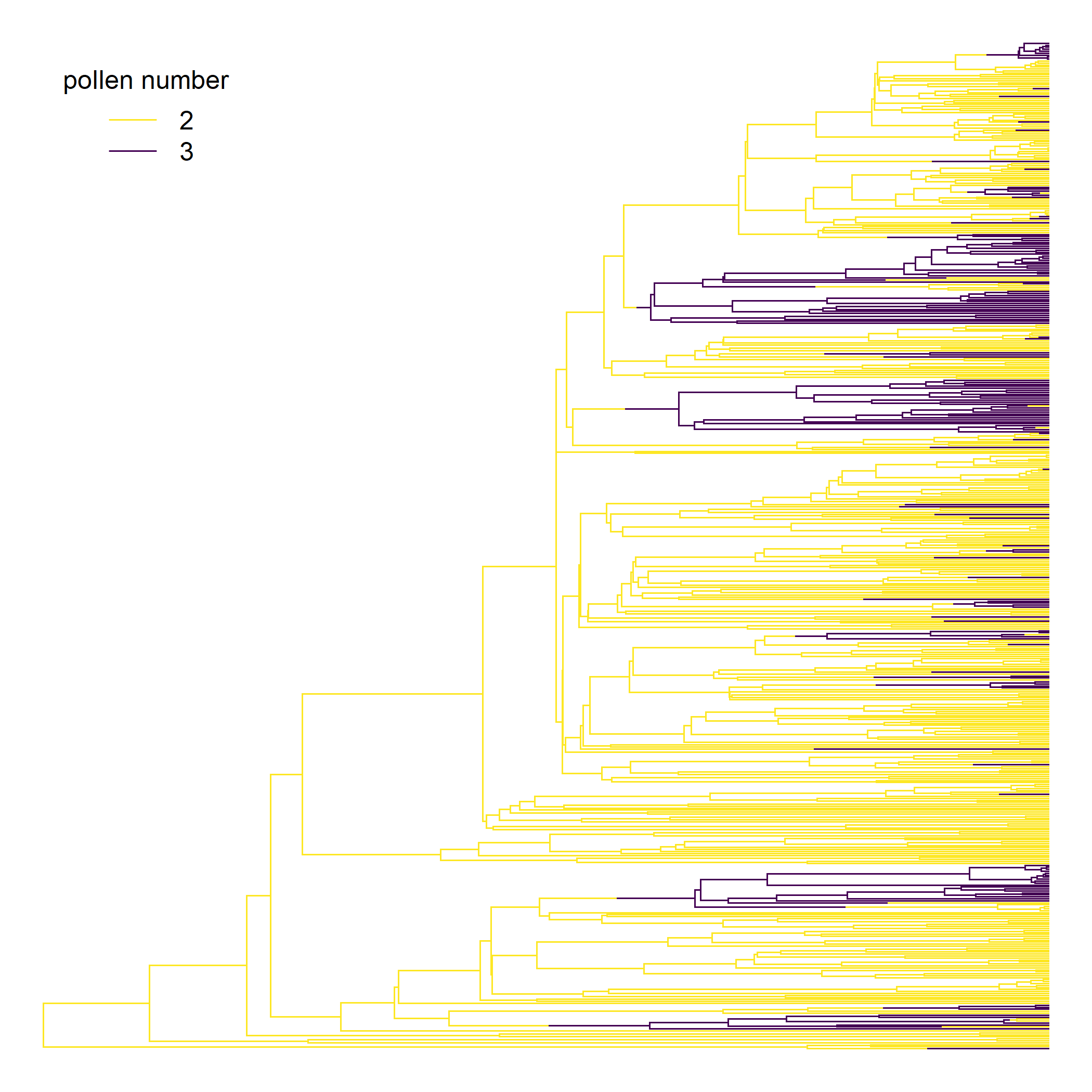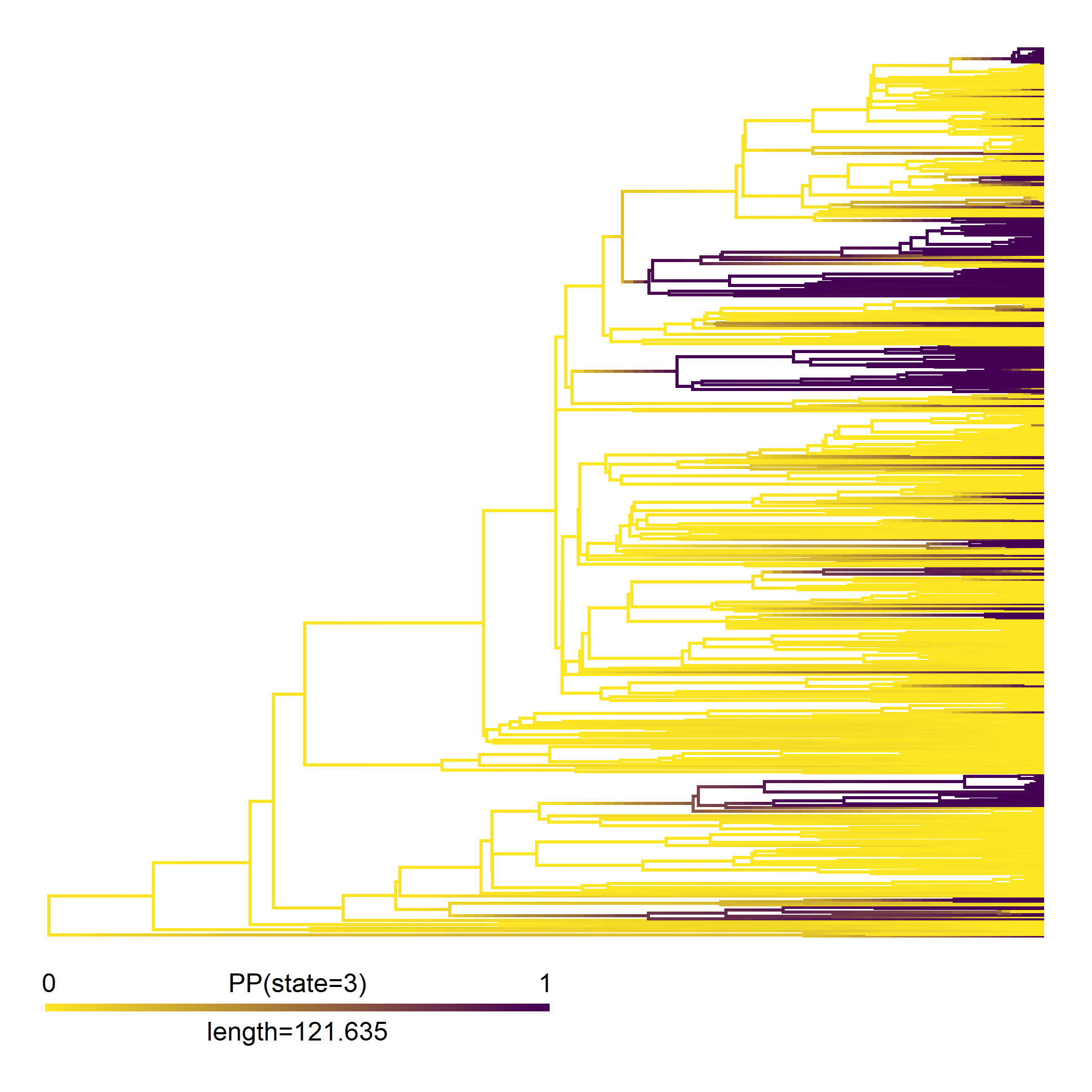## Tuesday, April 18, 2023

### Coloring the edges of a plotted tree by the marginal reconstructions part II: stochastic mapping

A short time ago, I posted a solution illustrating how to color the edges of the tree based on the most likely state at the descendant node of each edge from marginal ancestral state reconstruction.

The original question, though, pertained to stochastic mapping – so I thought it might be interesting to illustrate that case as well.

For this example, I’ll use pollen grain number in a sample of 511 species from a study by Williams et al. (2014).

``````library(phytools)
pollen.tree
``````
``````##
## Phylogenetic tree with 511 tips and 510 internal nodes.
##
## Tip labels:
##
## Rooted; includes branch lengths.
``````
``````pollen.data<-read.csv(file="http://www.phytools.org/Rbook/7/pollen-data.csv",
row.names=1)
``````
``````##                        V1
## Nuphar_lutea            3
## Nuphar_pumila           2
## Austrobaileya_scandens  2
## Schisandra_propinqua    2
``````
``````pollen<-setNames(pollen.data\$V1,rownames(pollen.data))
``````

Let’s fit an ARD model and then do stochastic mapping. For stochastic mapping we’ll use the new generic method `simmap`.

``````ard_pollen<-fitMk(pollen.tree,pollen,model="ARD",pi="fitzjohn")
ard_pollen
``````
``````## Object of class "fitMk".
##
## Fitted (or set) value of Q:
##           2         3
## 2 -0.002997  0.002997
## 3  0.004727 -0.004727
##
## Fitted (or set) value of pi:
##        2        3
## 0.860551 0.139449
## due to treating the root prior as (a) nuisance.
##
## Log-likelihood: -215.278634
##
## Optimization method used was "nlminb"
``````
``````pollen_simmap<-simmap(ard_pollen)
pollen_simmap
``````
``````## 100 phylogenetic trees with mapped discrete characters
``````

First, here’s our full set of stochastic mapped trees.

``````cols<-setNames(c("#FDE725FF","#440154FF"),2:3)
par(mfrow=c(10,10))
plot(pollen_simmap,cols,ftype="off",lwd=1)
``````Now, let’s compute the frequencies with which each node of the phylogeny is encountered in each of the two states. We can do this using our `summary` method for the object class as follows.

``````pollen_ace<-summary(pollen_simmap)
pollen_ace
``````
``````## 100 trees with a mapped discrete character with states:
##  2, 3
##
## trees have 81.14 changes between states on average
##
## changes are of the following types:
##        2,3   3,2
## x->y 57.58 23.56
##
## mean total time spent in each state is:
##                 2            3    total
## raw  1.935222e+04 4995.0410031 24347.27
## prop 7.948418e-01    0.2051582     1.00
``````

Our marginal frequencies are stored in an element of our object called `ace`. Just as we did before, let’s bind this together with a matrix representation of our tip states, and then used this large matrix to color the edges of the tree.

``````allStates<-rbind(ard_pollen\$data[pollen.tree\$tip.label,],
pollen_ace\$ace)
edge.cols<-cols[apply(allStates,1,function(x)
which(x==max(x)))][pollen.tree\$edge[,2]]
plot(pollen.tree,edge.color=edge.cols,no.margin=TRUE,
show.tip.label=FALSE)
legend("topleft",legend=sort(unique(pollen)),
title="pollen number",col=cols,lty="solid",bty="n",
inset=0.05)
``````That worked.

Maybe, instead of showing our most likely marginal states by edge, we may want to split each edge, and assign the root-wise and tip-wise halves of those edges to the most-likely condition of the closest node.

There are multiple ways I can think of to do this, here’s one using `phytools::paintSubTree` and the phytools `"simmap"` object class.

``````map<-reorder(pollen.tree,"cladewise")
map<-paintSubTree(map,node=Ntip(map)+1,
state=names(which(pollen_ace\$ace[1,]==max(pollen_ace\$ace[1,]))))
for(i in 1:nrow(map\$edge)){
states<-sapply(map\$edge[i,],function(x,aa)
names(which(aa[x,]==max(aa[x,]))),
aa=allStates)
if(length(unique(states))!=1)
map<-paintSubTree(map,node=map\$edge[i,2],state=states,
stem=0.5)
}
plot(map,cols,ftype="off",lwd=1)
legend("topleft",legend=sort(unique(pollen)),
title="pollen number",col=cols,lty="solid",bty="n",
inset=0.05)
``````Lastly, of course we can also compute a `"densityMap"` object in phytools if our character is binary. This might be done as follows.

``````dmap_pollen<-densityMap(pollen_simmap,plot=FALSE)
``````
``````## sorry - this might take a while; please be patient
``````
``````dmap_pollen<-setMap(dmap_pollen,cols)
plot(dmap_pollen,lwd=c(2,5),ftype="off")
``````That’s it!